# Non-Fourier Heat Transfer Analysis of Functionally Graded Spherical Shells under Convection-Radiation Conditions

Document Type : Research Paper

Authors

Department of Mechanical Engineering, School of Engineering, Persian Gulf University, Bushehr 7516913798, Iran

Abstract

Non-Fourier heat transfer analysis of functionally graded (FG) spherical shells subjected to the radiative-convective boundary conditions at their inner and outer surfaces are presented. It is assumed that the material properties have continuous variations along the thickness direction. The incremental differential quadrature method (IDQM) as an accurate and computationally efficient numerical tool is adopted to discretize the governing differential equations in both the spatial and temporal domain. The fast rate of convergence behavior and accuracy of the method is investigated through different examples and also, the results are compared with those available in the open literature wherever possible. Comparison studies between the results of IDQM and Runge-Kutta method (RKM) are also performed to establish the superior computational efficiency of the IDQM with respect to RKM. After validating the present formulation and method of solution, the influences of different parameters on the temperature time history and temperature distribution of the FG spherical shells are investigated. It is shown that the radiation boundary conditions decrease the period of oscillation of temperature time history. Also, it is observed that by increasing the non-Fourier time constant, the non-dimensional temperature oscillatory behavior increases. On the other hand, the present study reveals that the IDQM, as an accurate and practically efficient method, can be used for the analysis of different problems in the fields of the oil and petrochemical engineering in the future.

Keywords

#### Full Text

1. Introduction

Functionally graded materials (FGMs) are one of the advanced inhomogeneous materials with spatial compositional variation of their constituents which results in smoothly and continuously varying thermo-mechanical material properties. Usually FGMs are formed by mixing two different materials such as ceramics and metals. The different micro-structural phases of FGMs have different functions and the overall FGMs attain the multi-structural status from their property gradation. For example, in FGMs made of ceramics and metals, the ceramic constituents are able to withstand high-temperature environments due to their better thermal resistance characteristics, while the metal constituents provide stronger mechanical performance and reduce the possibility of catastrophic fracture . The FGMs can provide protection of the other materials from chemical and physical interaction with their environments (i.e., corrosion and erosion). In addition, these materials can operate in the extremely harsh thermal environmental conditions while maintaining their structural integrity . Due to these special benefits, FGMs have received substantial attention in various fields of technology such as chemical industries, refineries and petrochemical plant equipments in the form of pressure vessels, oil reservoirs, boilers, etc. Hence, the accurate heat transfer analysis of spherical shells made of FGMs becomes essential for engineering design and manufacture.

There are different theories for transient heat conduction analysis of solids. Fourier heat conduction theory relates heat flux directly to the temperature gradient using thermal conductivity. The accuracy of Fourier heat conduction law is sufficient for many practical engineering applications. However, this theory cannot accurately explain conduction of heat caused by highly-varying thermal loading . The Fourier heat conduction theory also breaks down at very low temperatures and when the applied heat flux is extremely large. In addition, Fourier’s law results in an infinite speed of thermal wave propagation, which is physically unrealistic. To better explain heat conduction in solids, non-Fourier heat conduction theories have been developed.

In recent years, some researchers studied the heat transfer analysis of homogeneous and FG spherical shells based on non-Fourier (hyperbolic) heat conduction law [3-7]. Babaei and Chen  employed the non-Fourier heat conduction to investigate the temperature distribution in heterogeneous spherical shells. The transient responses of temperature and heat flux were presented for different nonhomogeneity parameters and normalized thermal relaxation constants. Moosai and Shirmohammadi  compared the Fourier and non-Fourier results for an isotropic spherical shell subjected to periodic heat flux using the separation of variables method. In another work, Moosai  obtained the axisymmetric two-dimensional temperature distribution in an isotropic spherical shell using the same method. Chen and Chen  used the hybrid Green's function method to present the non-Fourier heat conduction analysis in the spherical shells. Keles and Conker  studied the heat conduction in thick-walled FG cylinders and spheres with exponentially-varying properties based on non-Fourier law. The problems were solved analytically in the Laplace domain and the results obtained were transformed to the real time space using the modified Durbin's numerical inversion method.

In all of these works, the radiation heat transfer from the boundaries of the shell is neglected. But, this heat transfer phenomena can change the thermal behavior of the shell, especially, when it works in a high temperature environment or its boundaries have much greater temperatures than the surrounding media. Hence, it is essential to study the influence of this type of boundary heat transfer phenomena when one performs the thermal analysis of a FG structure which usually operates under such a thermal condition. On the other hand, this type of boundary condition is nonlinear in nature and approximate solutions are needed to solve these nonlinear equations.

In the present work, the influences of radiation heat transfer together with the convection heat transfer from the boundaries of FG spherical shells on the their thermal behavior are studied. In order to include the influences of heat wave propagation velocity and consequently the better simulation of the conduction heat transfer in the shell, the non-Fourier heat transfer equation is used. The thermal parameters of the system can vary in the shell thickness direction. As an accurate and numerically efficient method, the incremental differential quadrature method (IDQM) [8-10] is employed to discretize the heat transfer equation subjected to the nonlinear boundary conditions in both the spatial and temporal domain. The resulting nonlinear system of equations is solved using the Newton–Raphson method. Comparison studies with the Runge–Kutta method (RKM) are performed to establish the accuracy and less computational time of the adopted method with respect to the RKM. After validating the method, some parametric studies are carried out.

2. Governing equation and solution procedure

Consider an FG spherical shell of inner radius and outer radius as shown in Fig. 1. It is assumed that the material properties of the shell vary continuously and smoothly in the thickness direction r according to the power law distribution such that the inner surface of the sphere is ceramic rich and its outer surface is metal rich. Hence, a typical effective material property ‘P’ can be represented as,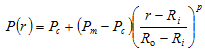(1)

where the subscripts m and c refer to the metal and ceramic constituents, respectively; p is the power law index or the material property graded index, which is a positive real number.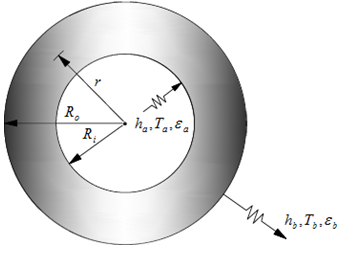Figure 1. The geometry of the functionally graded hollow sphere

It is assumed that the hollow sphere has an initial temperature distribution  and then suddenly exchange heat through its inner and outer boundaries with the mediums. The temperature of the inner and outer mediums are assumed to be constant and denoted as  and , respectively. Convection–radiation thermal conditions are assumed on the boundary surfaces of the FG shell.

Due to the axisymmetric initial and boundary conditions and also the material properties, the non-Fourier transfer equation in the absence of the internal heat generation can be written as,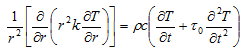(2)

where  is the temperature,  the thermal conductivity,  the mass density and  the specific heat capacity at an arbitrary material point of the sphere, respectively; also, the t and  are time and non-Fourier time constant (relaxation time), respectively.

The dimensionless form of heat transfer equation (3) can be expressed as,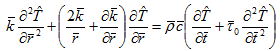(3)

where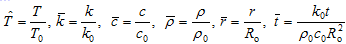(4)

,  and  are typical values of the thermal conductivity, the mass density and the specific heat capacity, respectively.

The non-dimensional initial and boundary conditions are as follows,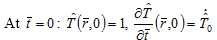(5)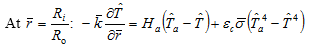(6)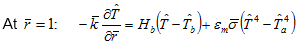(7)

where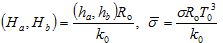(8)

, () and () refer to Boltzmann constant, convective heat transfer coefficient inside (outside) media of the shell and emissivity coefficient of the inside (outside) surface of the shell, respectively.

Due to the fact that Eq. (3) have variable coefficients and also nonlinear boundary conditions, it is difficult to solve it analytically. Hence, an appropriate numerical method should be employed to find the temperature distribution within the shell. In this work, the incremental differential quadrature method (IDQM) as an accurate and efficient numerical tool [8-10] is adopted. According to this method, the shell is discretized into a set of  grid points along the r-direction and the spatial derivative in heat transfer equation (3) is discretized at the domain grid points. The boundary conditions are exactly discretized at the boundary grid points. In order to discretize the temporal derivatives, the total time of interest is divided intosub-intervals or increments. Then, each temporal increment is discretized intodiscrete grid points and the temporal derivatives are discretized according to the DQM. In the following, a brief review of the basic formulation of this procedure is presented.

According to the IDQM, at a given grid point with and, the first and second-order derivatives of an arbitrary function with respect to the spatial and temporal variables are approximated as, respectively,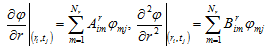(9.a, b)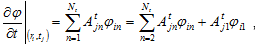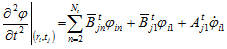(10.a, b)

where  is the weighting coefficients of the first-order derivative with respect to variable ; and are the weighting coefficient and the modified weighting coefficient of the second-order derivative with respect to variable r and t, respectively;  and  are the initial conditions; also hereafter, a dot over a variable means its first time derivative. It can be seen from Eq. (10) that the initial conditions are directly implemented into the discretized form of the function.

In order to determine the weighting coefficients, a set of test functions should be used in Eqs. (9) and (10). For polynomial basis functions DQM, a set of Lagrange polynomials is employed as the test functions [8-10]. The normalized weighting coefficients for the first and second-order derivatives in the r-direction are thus determined as, respectively,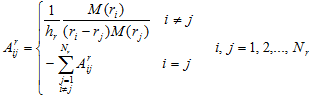(11)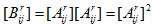(12)

where  and . In a similar manner, the temporal weighting coefficients can be obtained. In the numerical computation, Chebyshev-Gauss–Lobatto quadrature points are used for both spatial and temporal domains, that is,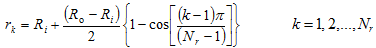(13)

Based on the DQ discretization rules, the DQ discretized form of the governing equation (3) can be written as,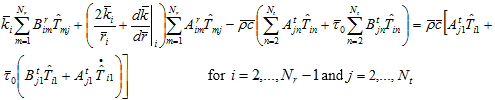(14)

In a similar manner, the external boundary conditions can be discretized. Finally, one obtains a system of nonlinear algebraic equations in each time interval. In this work, this system of nonlinear algebraic equations is solved using Newton–Raphson method and the procedure is repeated for all time intervals. At the end of each time interval, the temperature is used as the initial condition for the next time interval.

3. Results and analysis

In this section, after validating the present formulation and method of solution, some parametric studies are carried out. The FG spherical shells under consideration are assumed to be composed of zirconia (ceramic) and stainless steel (metal). The inner surface of the shells is ceramic rich and their outer surface is metal rich. The material constants for zirconia and stainless steel are given in Table 1.

 Table 1. Material properties of ceramic (zirconia) and metal (stainless steel). Material Stainless steel 12.1425 8166 390.3507 Zirconia 1.7752 5700 529.2691

The material properties in Eqs. (4) and (8) (i.e., ,  and ) are those of stainless steel. Also, otherwise specified, the convective-radiative boundary conditions at the inner and outer surfaces of the shells is considered and, the following numerical values for the thermal and geometrical parameters are used,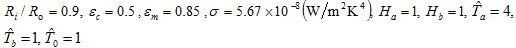As a first example, the convergence behavior of the presented IDQM against the number of spatial grid points are investigated in Table 2. The fast rate of convergence of the method is quite obvious. Also, one can observe that seventeen grid points  is sufficient to obtain the quite acceptable results and, hence, hereafter this number of grid points is used to extract the numerical results.

 Table 2. The convergence behavior of the method against the number of spatial grid points (,, ). 11 1.8052 2.4387 2.5537 13 1.8007 2.4842 2.5384 15 1.7900 2.4978 2.5384 17 1.7968 2.4932 2.5517 19 1.7968 2.4932 2.5517 21 1.7968 2.4932 2.5517

The effects of number of temporal sub-domain and grid points per temporal sub-domain on the results are studied in Table 3. Also, the results obtained using the Runge-Kutta method are reported in this table. Again, the fast rate of convergence of the IDQM can be observed. Also, it can be seen that using the IDQM, accepatble results can be obtained using a few number temporal sub-domains and grid points per temporal sub-domain. In addition, faster rate of convergence of the IDQM with respect to the Runge-Kutta method is quite evident. In this table, a comparison between the CPU time requirements of these two methods is also made, which shows much less computational efforts of the IDQM with respect to the Runge-Kutta method. Furthemore, the IDQM and Runge-Kutta results for the time history of the non-dimensional temperature at  are compared in Fig. 2. The comparison studies are performed for the two types of boundary conditions. One can see from the data presented in Table 3 and Fig. 2 that excellent agreement exists between the results of the IDQM and RKM. Based on the obtained data in this eaxmple, hereafter a value of and are used to obtain the numerical results.

In order to further demonstrate the accuracy of the method of solution, non-Fourier heat conduction of a homogeneous spherical shell subjected to two different types of boundary conditions, for which analytical solution were obtained by Chen and Chen , is considered. It should be mentioned that due to its non-homogeneous essential boundary conditions, this is a challenging problem for the numerical methods. The initial and boundary conditions are taken as follows,

Initial conditions: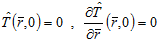(15)

Boundary conditions: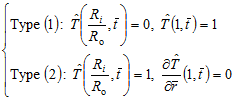(16.a, b)

The results for the temperature distribution within the shells subjected to the both types of boundary conditions and at different non-dimensional times are presented in Fig. 3. It can be seen that the method predicts the thermal wave propagation in the shell close to the analytical solution  for both types of boundary conditions.

 Table 3. The convergence behavior against the number of temporal sub-domain and grid points per temporal sub-domain and comparison study with Runge-Kutta method. Method CPU-time (s) IDQ 10 17 1.7963 2.4929 2.554 0.4975 19 1.7959 2.4936 2.5525 0.5977 21 1.7963 2.4935 2.5518 0.7292 15 17 1.7966 2.4934 2.552 0.6538 19 1.7968 2.4932 2.5517 0.7842 21 1.7967 2.4932 2.5512 0.9529 45 17 1.7966 2.4932 2.5512 1.3722 19 1.7966 2.4932 2.551 1.7691 21 1.7966 2.4932 2.5511 2.0754 Runge-Kutta 1.7977 2.4931 2.5504 302.735 1.7969 2.4932 2.5508 1078.717 1.7968 2.4932 2.5509 1790.6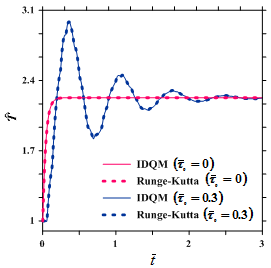(a) Convective boundary condition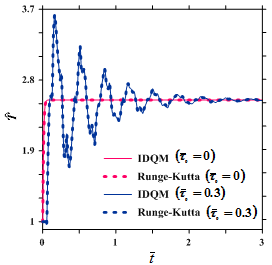(b) Convective-radiative boundary condition

Figure 2 (a), (b).  Comparison between the IDQM and Runge-Kutta results for the non-dimensional temperature time history at .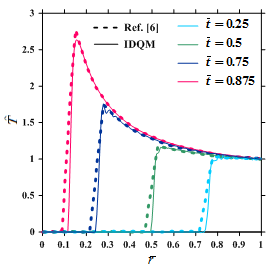(a) Type (1) (Eq. (16.a))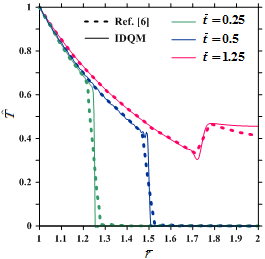(b) Type (2)(Eq. (16.b))

Figure 3 (a), (b). Comparison between the non-dimensional temperature distributions for the spherical shell subjected to the different types of boundary conditions as given in Eqs. (16.a, b).

After validating the present formulation and method of solution, some parametric studies are carried out. As a first case, the influences of boundary condition on the temperature time history and temperature distribution of FG spherical shells are investigated in Figs. 4-6. In these figures, the results for the shells with convective-radiative, only convective and only radiative boundary conditions at the inner and outer surfaces are campared. It can be observed that the heat transfer via the radiation phenomena is not negligible. But, in the most previous studies on heat conduction analysis of the FG shells, this heat transfer phenomena is not considered.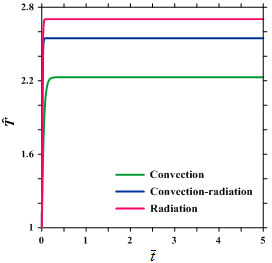(a) Fourier law ()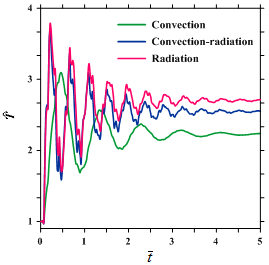(b) Non-Fourier law ()

Figure 4 (a), (b). The influences of the boundary conditions on the non-dimensional temperature time history based on Fourier and non-Fourier law at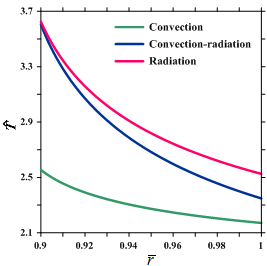(a) Fourier law ().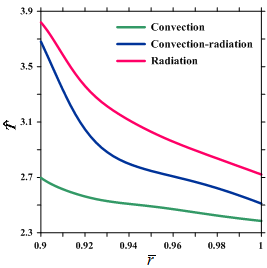(b) Non-Fourier law ().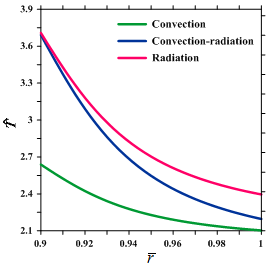(c) Fourier law ().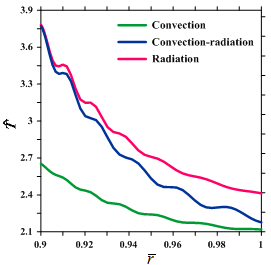(d) Non-Fourier law ().

Figure 6 (a)-(d). The influences of the boundary conditions on the non-dimensional temperature distribution at two different time level.

The effect of the material graded index (p) on the temperature distribution across the thickness of the FG spherical shell is shown in Fig. 7. One can see that material graded index (p) has significant effect on the temperature distribution of the shell. Also, it is obvious that by increasing this parameter, the results approach to those of a shell made of zirconia.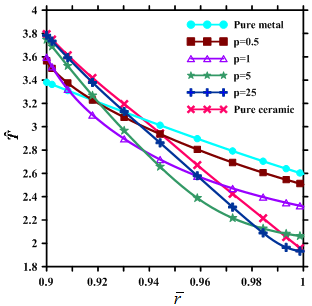Figure 7. The effect of material graded index (p) on the non-dimensional temperature distribution across the shell thickness .

The influence of relaxation time constant on the time history of the non-dimensional temperature at the surface  is shown in Fig. 8 for the FG shells subjected two types of boundary conditions. It is clear that by increasing the relaxation time value, the non-Fourier effect increases and, consequently, the non-dimensional temperature oscillatory behavior raises as well. In all cases, for , the non-Fourier effects is alomost damped. In addition, it is observed that for a given value of the time constant, the raditve heat transfer phenomena, decreases the period of the oscilation.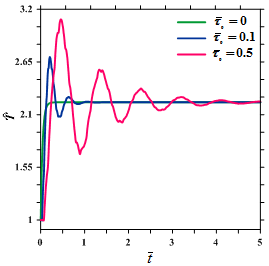(a) Convective boundary condition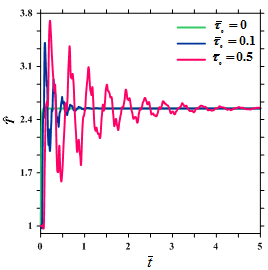(b) Convective-radiative boundary condition

Figure 8 (a), (b). The effect of relaxation time on the non-dimensional temperature time history of the surface .

4. Conclusion

In this paper, the IDQM was employed to study the heat transfer analysis of FG spherical shells based on the non-Fourier heat transfer law under convective-radiative boundary conditions. Differential quadrature method was employed successfully to discretize the spatial and temporal domains with no restriction on the time steps and temporal and spatial grid spacing to avoid the numerical instability for this challenging nonlinear problem. It was shown that the converged accurate results were obtained with less computational time efforts with respect to the Runge-Kutta method and thus, reducing the computational cost. After validating the formulation and method of solution, the effects of different types of thermal boundary conditions were investigated. It was shown that the radiative heat transfer phenomena have significant effect on the time history of temperature and temperature distribution within the FG spherical shells. Also, it is found that by considering this type of boundary condition, the period of temperature time history oscillation decreases. As another important parameter, the influence of relaxation time on the time history of the non-dimensional temperature was studied and it was shown that by increasing the value of this parameter, the non-dimensional temperature oscillatory behavior raises as well. Finally, the potential of the IDQM as an accurate and practically efficient method for a challenging problem was explored. This method may be used for the analysis of different problems in the fields of the oil and petrochemical engineering in the future.

//

#### References

       Shen HS. Functionally Graded Materials. Taylor & Francis Group 2009.
       Babaei MH, Chen ZT. Transient hyperbolic heat conduction in a functionally graded hollow cylinder. International Journal of Thermophysics 2007; 24:325-30.
       Babaei MH, Chen ZT. Hyperbolic heat conduction in a functionally graded hollow sphere. International Journal of Thermophysics 2008; 29:1457-69.
       Shirmohammadi R, Moosai A. Non-Fourier heat conduction in a hollow sphere with periodic surface heat flux. International Communications in Heat and Mass Transfer 2009; 36:827-33.
       Moosai A. Axisymmetric non-Fourier temperature field in a hollow sphere. Journal of Applied Mechanics 2009; 79:679-94.
       Chen TM, Chen CC. Numerical solution for the hyperbolic heat conduction problems in the radial-spherical coordinate system using a hybrid Green's function method. Journal of Thermal Science 2010; 49:1193-6.
       Keles I, Conker C. Transient hyperbolic heat conduction in thick-walled FGM cylinders and spheres with exponentially-varying properties. European Journal of Mechanics A/Solids 2011; 30:449-55.
       Malekzadeh P, Rahideh H. IDQ two-dimensional nonlinear transient heat transfer analysis of variable section annular fins. Energy Conversion and Management 2006; 48:269-76.
       Rahideh H, Malekzadeh P, Golbahar Haghighi MR. Heat conduction analysis of multi-layered FGMs considering the finite heat wave speed. Energy Conversion and Management 2012; 55:14-9.
     Malekzadeh P, Fiouz AR, Razi H. Three-dimensional dynamic analysis of laminated composite plates subjected to moving load. Composite Structures 2009; 90:105-14.